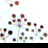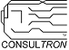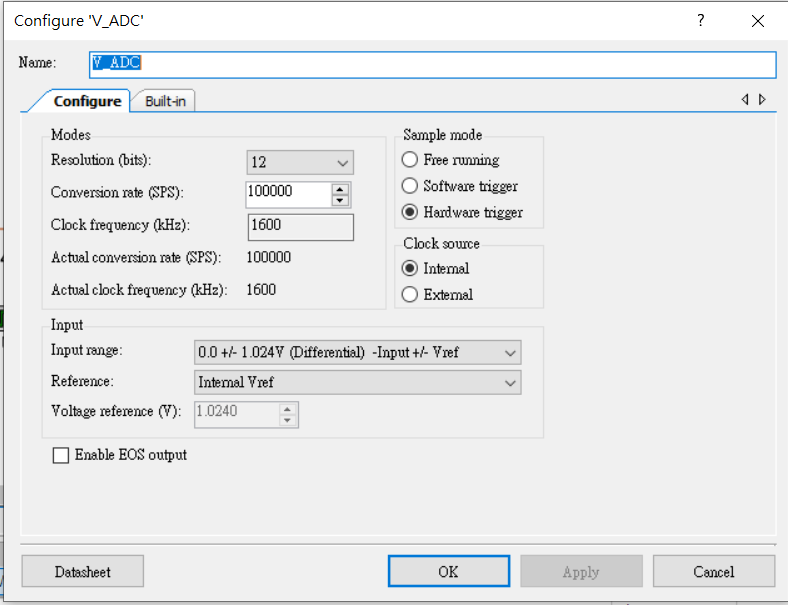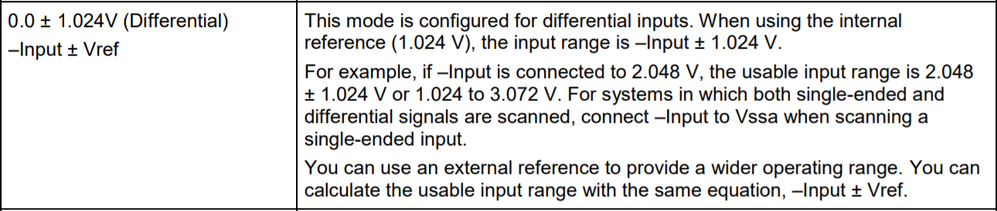cancel
Showing results for
Did you mean:

PSoC™ 5, 3 & 1New Contributor II

psoc 5lp provide voltage and adc component question

hello ,

i have two situations and some questions show below.

a)i have a project need to measure AC voltage and current.both of voltage and current sensors Vout have dc offset which depend on how much power source i give them.

i connect CY8CKIT-059 to my computer through usb port and i measure its Vdd,it shows 4.6V.so i provide this Vdd to my sensor then sensor's dc offset goes to 2.3V at AC 0V.

but when i disconnect usb port and connect it again,Vdd will be changed to 4.52V sometimes will be 4.38 or 4.44V.it will cause dc offset change to 2.25V or 2.19V at AC 0V.

1.could someone tell me why Vdd is not stable?

b)i convert adc value to mV,the value should be 2300V at 0V.unfortunately there are some noise to cause value unstable(value would be 2324,2285,2292...).

i have gone through datasheet and don't understand description that i marked.

1.do that mean if i want to filter the noise,i can connect sensor Vout to P0 or P0?

2.if i choose Internal Vref,bypassed mode,i can't choose those as analog high-z pins.is that right?

3.what if i set Internal Vref,bypassed mode and higher conversion rate,will the value be more stable than Internal Vref mode?

all i want to do is filter noise and eliminate error as much as i can.but i have no idea about it.

sean

1 SolutionHonored Contributor II

Re: psoc 5lp provide voltage and adc component question

sean,

In general, I never try to use VDD (actually VDDA) as a Vref to ADCs.   This is because if I use the USB power, it can vary too widely.  The differences you are seeing with VDD measurements can be for multiple reasons.  The USB 5V feed can vary from the host (4.75V to 5.5V).  Then there is a 0.1V to 0.3V through the schottky diode (D1) on the KitProg board.  If the load on the CY8CKIT-059 varies, this will cause the schottky diode to drop more voltage and any series resistance in the VDD circuit all the way from the host to see more voltage drop.

I believe the safest practice is to use the bandgap voltage source (1.024V) as a Vref to the ADC.  This voltage source is mostly immune to VDD fluctuations.  Even if VDD drops to 1.8V, the bandgap should be stable.  Additionally, the bandgap source has a tigher tolerance for voltage regulation.  Usually better than most external regulators.

Len

Len
"Engineering is an Art. The Art of Compromise."
10 RepliesHonored Contributor II

Re: psoc 5lp provide voltage and adc component question

sean,

In general, I never try to use VDD (actually VDDA) as a Vref to ADCs.   This is because if I use the USB power, it can vary too widely.  The differences you are seeing with VDD measurements can be for multiple reasons.  The USB 5V feed can vary from the host (4.75V to 5.5V).  Then there is a 0.1V to 0.3V through the schottky diode (D1) on the KitProg board.  If the load on the CY8CKIT-059 varies, this will cause the schottky diode to drop more voltage and any series resistance in the VDD circuit all the way from the host to see more voltage drop.

I believe the safest practice is to use the bandgap voltage source (1.024V) as a Vref to the ADC.  This voltage source is mostly immune to VDD fluctuations.  Even if VDD drops to 1.8V, the bandgap should be stable.  Additionally, the bandgap source has a tigher tolerance for voltage regulation.  Usually better than most external regulators.

Len

Len
"Engineering is an Art. The Art of Compromise."New Contributor II

Re: psoc 5lp provide voltage and adc component question

len,

i'm not sure what you mean because my bad comprehension.

1.did you mean use Vref component as a input connect to adc negative input(here's an attach show below)?if so,which differential mode should i choose?

2.did you mean use Vref component as a source voltage provide to my sensor?Honored Contributor II

Re: psoc 5lp provide voltage and adc component question

sean,

Definitiely 1.   I don't know how familiar you are with ADC theory of operation.  In short, the ADC output is "ratiometric" to the Vref supplied.  For example, the Vref is 1.024V, the full scale range is 0V to 1.023V and the ADC resolution is 10 bits.  With a 10 bit resolution you have 1024 ADC counts.  These counts are divided evenly across the range.  Therefore each ADC Count = 1mV (in this example). Therefore

If you use VDDA as your Vref, then ADCcount = Vin*(2^ADCres)/VDDA.  If VDDA varies as you indicated, then the ADCcount will vary even if Vin remains constant.

Using the Bandgap voltage source as Vref is mostly immune to changes in VDDD and VDDA.  Therefore it is considered more stable.

Len

Len
"Engineering is an Art. The Art of Compromise."New Contributor II

Re: psoc 5lp provide voltage and adc component question

len,

i recently created a new project and did the way what you said.but there are some problems confuse me.

according to datasheet,it said the input range is -input ± 1.024 when using the internal reference.

if i connect 1.024V to negative input,input range will be 0 to 2.048V.when Vin is GND,adc count is -2035

and unstable as well.when Vin is Vdd,adc count is 2047 and very stable.

1.why is adc count not 0 when Vin is connected to GND?

2.if Vin is greater than 2.00V,adc count is always 2047.how come?if this is because the maximum input is 2.048V,why does it happen at 2.00V rather than 2.048V?

attach show below are adc configuration and datasheet.Honored Contributor II

Re: psoc 5lp provide voltage and adc component question

sean,

 1.why is adc count not 0 when Vin is connected to GND?

I assume the Vin listed in your question is connected to the '+' input terminal of the ADC (ie. "+Input").

What is the '-' input terminal connected to?

In differential mode the "-Input" referred to is the Input voltage of the '-' terminal of the ADC to VSSA.   Therefore, if -Input is set to 1.00V (referenced to VSSA) and Vin (+Input) is 0V (referenced to VSSA) your differential result (Vdiff) = -1.00V.  The ADC count with be (Vdiff/Vref) * 2^11 = (-1.00V/1.024V) * 2^11. = -0.976*2048 = 1999 ADC counts

 2.if Vin is greater than 2.00V,adc count is always 2047.how come?if this is because the maximum input is 2.048V,why does it happen at 2.00V rather than 2.048V?

• Is Vin the differential input voltage in the (ie. +Input - -Input)?
• If Vin is the voltage from +Input to VSSA, what is the voltage from -Input to VSSA?

Len

Len
"Engineering is an Art. The Art of Compromise."New Contributor II

Re: psoc 5lp provide voltage and adc component question

len,

1.

when Vin is GND:

i calculated adc count by formula as you said is (Vdiff/Vref) * 2^12 = (-1.024/2.048) * 4095 = -2047

there is about 20 offset between them.is it normal?

when Vin is Va:

adc count = (Vdiff/Vref) * 2^12 = ((1.15-1.024)/2.048) * 4095 = 251

there is about 150 offset between them.are there something wrong that i don't know or misunderstand?

2.

if Va is greater or equal to 2V,adc count will always be 2047.

3.

is that mean i use Vdda as Vref to adc if i select input range to –Input ± Vdda?Honored Contributor II

Re: psoc 5lp provide voltage and adc component question

sean,

 3. is that mean i use Vdda as Vref to adc if i select input range to –Input ± Vdda?

If you use VDDA as Vref you have the same issue mentioned in my earlier post.  The ADC counts are ratiometric to VDDA which can change depending on a number of factors including load.  Therefore my recommendation is still to use the bandgap as Vref.

I've attached a modified version of your original project.  I changed the ADC component to be Vref from the bandgap with a 0 to 2.048V range.  It will match the V_ADC component for range.   Also, I placed the read() inside the for() loop with a delay of 1 second between reads.  Therefore you shouldn't need to reset your PSoC to get a new ADC reading set.

 1.when Vin is GND:i calculated adc count by formula as you said is (Vdiff/Vref) * 2^12 = (-1.024/2.048) * 4095 = -2047i got adc count from V_ADC_GetResult16() is -2024.there is about 20 offset between them.is it normal?

Yes.  All ADCs circuits have some potential gain and offset errors.  I usually go through a calibration sequence after the build to nullify these errors if the measured return value is needed to be that accurate.

 2.if Va is greater or equal to 2V,adc count will always be 2047.

If the input is at or above upper value of the range, the ADC count should be the maximum value.  In the case of a 12-bit ADC in differential mode it is (2 to the power of 11) -1 which is 2047.   In your case, the 2V at Vin is close to the upper value of the range.  Probably because of gain and offset errors, the 2V appears to be 2.048V (or higher) to the V_ADC.

Len

Len
"Engineering is an Art. The Art of Compromise."New Contributor II

Re: psoc 5lp provide voltage and adc component question

len ,

if variable resistor 'R' in the top design is a current sensor and its output Va is depended on how much current through the load.if this sensor scale factor is 1A : 1Va and can measure current up to 5A,then if i have 5A  through the load,Va will be 5V.

now the problem occurs.if i use the method as you recommendated,i only can measure Va from 0 to 2.048V and current as well.greater than 2.048 will always be 2.048  that i measure.

(by the way,i still confuse why there is about 150 offset of adc count when Va is at 1.15V.)Honored Contributor II

Re: psoc 5lp provide voltage and adc component question

sean,

I recommend you change your current sensor scaling to have 5A = 2.048V.   The easiest method is to scale your input voltage to match the ADC range.

Remember, you haven't corrected for gain or offset.   Are you sure you are measuring 1.15V?  What is the calibrated accuracy of your meter?  Are you using an oscilloscope for this measurement?

Remember, you are using VDD as your 4.53V source.   Is VDD bouncing with load changes?

Len

Len
"Engineering is an Art. The Art of Compromise."Honored Contributor II

Re: psoc 5lp provide voltage and adc component question

sean,

Attached a modified version of your test program if you're interested.

I removed the LCD calls ( I don't have one to use).  I replaced the LCD calls with a UART terminal for outputs and inputs.

When you run the program, you enter a number from 0 to 255 (Enter the TARGET VDAC value  (0 to 255) => ) .  It sends it to a VDAC with a 0 to 4.08V range to set the output value.  This VDAC is directly connected to Vin.

Using a calibrated meter, enter the voltage output read (Enter the meter MEASURED input voltage => ) on the Vin pin (P3.4).